# Queues

### Overview

As we saw, stacks are "Last In First Out" (LIFO) data structures. This means that the last thing added to the stack is the first thing that is removed.

If we do the opposite of this, and remove the first thing we added instead of the last, then we get a queue. A queue is a "First In First Out" (FIFO) data structure.

You can think of a queue as a list of objects waiting in line. Queues always have a start and an end. Objects are added at the end of the queue, and removed from the start. The following image depicts a queue.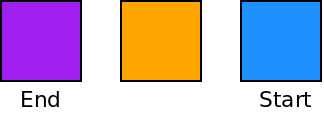If we want to add to the queue, we must add to the end. This operation is called "enqueue".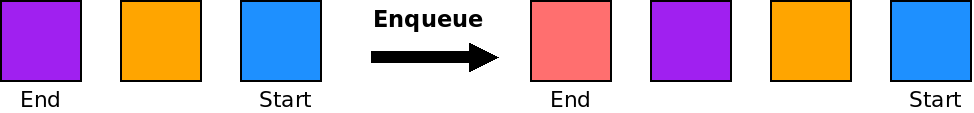Likewise, if we want to remove an element from a queue, we have to do so from the start. This operation is called "dequeue".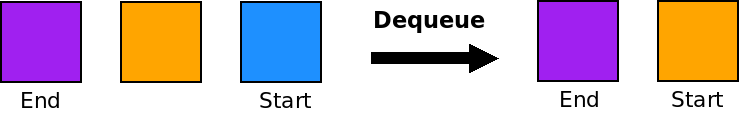### Applications of Queues

Queues are useful whenever we want to store things in order and get them back out in the same order:

• Buffering data.
• Output.
• Inter-Process Communication.
• Networking.
• Simulating lines.
• Handling multiple clients (servers).

### Implementation with an Array

Like stacks, queues can be implemented using either an array or a linked list. Since queues add at one end, and remove from the other, they are a little more complicated to implement than stacks.

In our array, we have to keep track of the start and the end. Initially, we start both of these at negative one as the queue is empty: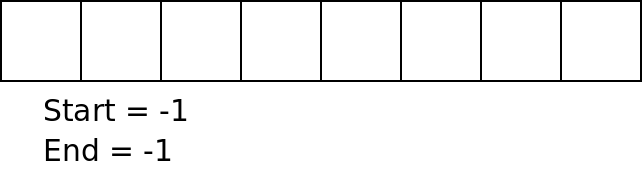When we enqueue elements, we add them at the end, and increment the end variable: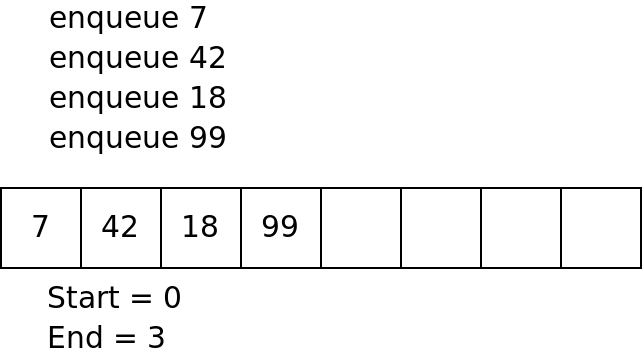Then, when we dequeue elements, we take them from the start and increment the start variable: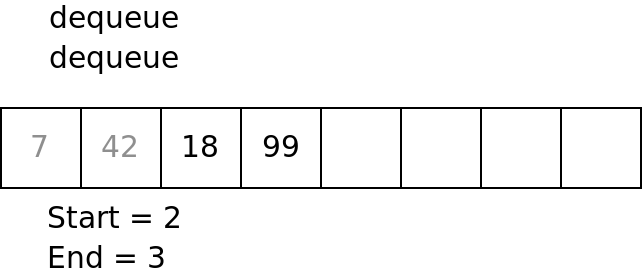The tricky part of implementing a queue with an array, is that the start and then end can both wrap around the end of the array. If we enqueue 4 more things in the example above, we will need to loop the end back to 0.

This example shows a queue implemented with an array.

### Implementation with a Linked List

Queues can also be implemented with a linked list. Because queues require us to access both ends of a data structure, this would be done most efficiently with a doubly-linked list.

The enqueue function would simply add an element at one end of the linked list, and the dequeue function would remove an element from the other end.

One common algorithm that is implemented using a queue is breadth-first search or BFS. Imagine we have a rectangular maze consisting of either wall or empty space. We want to go from the start of the maze which is the upper left corner, to the end which is the bottom right.

In a program, we can represent such a maze with a two-dimensional array of characters like so:

  char maze  = {
"-#################",
"----------##-----#",
"-########-##-##-##",
"-########----##-##",
"-########-#####-##",
"-----####-#####-##",
"#########-###---##",
"####------#####---"};


To get from the start to the end, we will start at the start location, and explore out from there until we reach the end location.

In order to keep track of where we need to go next, BFS uses a queue. Locations that we need to visit are enqueued and then when we need to move, we dequeue a position and go there.

In order to avoid going back to the same place multiple times, we need to mark the locations that we have already visited. Pseudocode for this algorithm is:

1. Set current to the start position.
2. While current is not the end position:
1. Mark current as visited.
2. If we haven't gone left, enqueue left.
3. If we haven't gone right, enqueue right.
4. If we haven't gone up, enqueue up.
5. If we haven't gone down, enqueue down.
6. If the queue is empty, there is no path!
7. Set current to dequeue( ).
3. We made it!

This example shows how BFS can be used to solve the maze given above.

Breadth-first search is called "breadth-first" because it does a broad scan. It checks multiple paths step by step rather than going fully down one path. For example, when we get to the fork at (0, 1), BFS will follow both paths by looking at (1, 1) then (0, 2) then (2, 1) then (0, 3) and so on. It works this way because the queue stores the places in the order we get to them.

An alternative way to solve this maze is using depth-first search, DFS. This works almost exactly the same way as BFS, except that instead of using a queue to hold the locations to search, we use a stack instead.

When we use a stack, we will visit the locations in the maze in a different order. This example shows DFS in action.

This algorithm is called "depth-first" because it follows each path as far as it can before leaving it. For example, when we get to the fork at (0, 1), DFS will follow the downward path until it reaches a dead end before returning back up to (1, 1). If we had rearranged the pushes so that down came before right, DFS would never have gone down that path at all.

### Priority Queues

For some applications, we want to handle things roughly in order but may have some exceptions. For example, in an operating system scheduler, processes are given time on the CPU roughly in order, but some high priority processes can preempt others.

A queue that keeps some notion of priority in this manner is called a priority queue.

There are several ways to implement priority queues. One is to have a priority along with each object. Then on a dequeue, you would scan through looking for the highest priority item and remove it. This is clearly slower than a normal dequeue operation. Another way is to maintain separate queues for each priority level. We will see other solutions for this problem later in the semester.